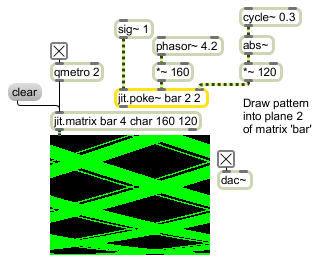jit.poke~

Write an audio signal into a matrix

Description

The jit.poke~ object writes the value specified by the leftmost signal input into one plane of a matrix cell at the position specified by the signal inputs (following the leftmost input). The object arguments are [matrix_name] [dim_inputcount] [plane] .

Arguments

Name Type Opt Description
matrix_name The name of the matrix to access (default = none)
dim_inputcount The number of dimensions in the matrix (default = 2)
plane The plane in a cell whose value is replaced by leftmost input (default = 0)

Messages

 signal In left inlet: Signal values arriving in this inlet will be stored at the dimensional locations specified by the signal inputs to the remaining inlets of the jit.poke~ object. The number of dimensional inlets will vary, depending on the value of the [dim_inputcount] argument. In other inlets: Signal values arriving in this inlet specify the dimensional location of the data to be stored for the plane specified. A signal inlet is provided for each dimension specified by the [dim_inputcount] attribute (i.e., jit.poke~ bigcube 3 has 4 inlets -- one for input data, and one for each of the 3 dimensions of the matrix).

Attributes

Name Type g/s Description
matrix_name symbol The name of the matrix to access (default = none)
normalize int Normalized input coordinates flag (default = 0)

Examples